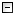Home Control Panel Login Register Search Submit Logout About me Contact meMy ResearchWeb This Site
 Home > My Research > SAS GCM power > SAS power analysis Macros for a quadratic growth curve model
SAS power analysis Macros for a quadratic growth curve model
2009-04-16    Zhang, Z. & Wang, L.       Read: 12775 times
Cite this page: Zhang, Z. & Wang, L. (2009). SAS power analysis Macros for a quadratic growth curve model. Retrieved March 22, 2023, from http://www.psychstat.org/us/article.php/81.htm.
 SAS Macros for a quadratic growth curve model These macros are licensed under the GNL General Public License Version 2.0. You can use/modify/distribute those macros. For questions or comments, please contact Johnny Zhang at zhiyongzhang(@)nd.edu. It would be appreciated if you cite the macros in the following way:Zhang, Z., & Wang, L. (2009). Power analysis for growth curve models using SAS. Behavior Research Methods, 41(4), 1083-1094. Request a copy /*Suppress the output and the log */options nosource nonotes nosource2 nomprint; /*Power analysis of growth curve models using SAS*//*CHANGE THE PARAMETERS HERE*/*model parameters;%LET MuL=10;        *mean level/initial status;%LET MuS=.1;       *mean slope/rate of change;%LET MuQ=.1;       *mean acceleration;%LET Sigma_e=1;                *residual standard deviation;%LET Sigma_L=1;                 *level standard deviation;%LET Sigma_S=1;                *slope standard deviation;%LET Sigma_Q=1;          *quadratic term standard deviation;%LET rho_LS=0;              *correlation between levels and slopes;%LET rho_LQ=0;              *correlation between levels and accelerations;%LET rho_SQ=0;              *correlation between slopes and accelerations;%LET miss=0.1;       *missing data rate, 0: no missing data;*power parameters;%LET R=5000;       *number of simulation replications;%LET T=6;          *number of measurement occasions;%LET start=500;     *the minimum sample size to consider;%LET end=500;     *the maximum sample size to consider;%LET step=100;      *the step between two sample sizes;%LET df=4;         *the difference in the numbers of parameters;%LET seed=4321;     *random number generator seed; /*DO NOT CHANGE CODES BELOW UNLESS YOU KNOW WHAT YOU ARE DOING*/ /*The first Macro: LL*//*Calculate the chi-square difference of two nested growth curve models*/%MACRO LL(N,T,seed);DATA Sim_QuaGM;* set statistical parameters;  N = &N; seed = &seed;* setup arrays so that we can include multiple variables for repeated measures;  ARRAY y_score{&T} y1-y&T;  ARRAY M{&T} m1-m&T;  m1=1;* generate raw data with considering the missing data rate;  DO _N_ = 1 TO N;    e_L=RANNOR(seed);    e_S=&rho_LS*e_L+SQRT(1-&rho_LS**2)*RANNOR(seed); e_Q=&rho_LQ*e_L+(&rho_SQ-&rho_LS*&rho_LQ)/(1-&rho_LS**2)*(e_S-&rho_LS*e_L)            +sqrt(( (1-&rho_LS**2)*(1-&rho_LQ**2)-(&rho_SQ-&rho_LS*&rho_LQ) )/SQRT(1-&rho_LS**2) )*RANNOR(seed);    L_score=&MuL+&Sigma_L*e_L;    S_score=&MuS+&Sigma_S*e_S; Q_score=&MuQ+&Sigma_Q*e_Q;* include indicator variables to generate missing data;    DO t = 1 TO &T;      y_score{t} = L_score + (t-1) * S_score + (t-1)**2*Q_score+ &Sigma_e*RANNOR(seed);      END; DO t=2 TO &T;   m{t}=m{t-1};   IF m{t-1}=1 AND  RANUNI(seed) > (1-&miss * (t-1))/(1-&miss * (t-2)) THEN m{t} = 0;      IF m{t}=0  THEN y_score{t}=.;   END;    KEEP y1-y&T;    OUTPUT;    END;RUN; DATA QuaGM;  SET Sim_QuaGM;  %DO t = 1 %TO &T;    id = _N_; time=&t-1; time2=(&t-1)**2; y=y&t; OUTPUT;  %END;  KEEP id time time2 y;RUN;  /*Fit two nested models to the data*/ODS OUTPUT FitStatistics(persist=proc)=fit;*Model 1: the true model;TITLE1 'Quadratic Growth Model';PROC MIXED DATA=QuaGM NOCLPRINT COVTEST MAXITER=100 METHOD=ML;  CLASS id;  MODEL y = time time2 /SOLUTION DDFM=BW CHISQ;  RANDOM INTERCEPT time time2/ SUBJECT=id TYPE=UN GCORR;RUN; *Model 2: without the quadratic term;PROC MIXED DATA=QuaGM NOCLPRINT COVTEST MAXITER=100 METHOD=ML;  CLASS id;  MODEL y =  time  /SOLUTION DDFM=BW CHISQ;  RANDOM INTERCEPT time / SUBJECT=id TYPE=UN GCORR;RUN; ODS OUTPUT CLOSE;%MEND LL; /*The second Macro: POWER*//*This Macro calls the first Macro LL for each replication*/* Calculate power based on R replications; %MACRO POWER(R,N,T,seed,df);DATA tempfit;  DO _N_=1 TO 8;    tempfit=_N_; OUTPUT;  END;RUN;  %LL(&N,&T,&seed);DATA fit;  MERGE fit tempfit;RUN; DATA allfit;  SET fit;RUN; %DO I = 2 %TO  &R;  PROC DATASETS LIBRARY=WORK; DELETE fit; RUN; QUIT;  %LL(&N,&T,%eval(&seed+&I*1389));  DATA fit;    MERGE fit tempfit;  RUN;   DATA allfit;    SET allfit fit;  RUN;  DM 'CLEAR LOG';%END;  DATA allfit;  SET allfit;  IF MOD(_N_,4) ~= 1 THEN DELETE;  KEEP Value;RUN; DATA allfit;  SET allfit;  id =INT((_N_-.1)/2)+1;  modelnum = MOD(_N_+1, 2);RUN; PROC TRANSPOSE DATA=allfit OUT=allfit prefix=model;  BY id;  ID modelnum;  VAR Value;RUN; DATA allfit;  SET allfit;  ss = &N;  diff = model1 - model0;  ind = 1;  IF diff < CINV(.95, &df) THEN ind = 0;  DROP id _NAME_ model0 model1;RUN; PROC MEANS DATA = allfit;  VAR ss ind;  OUTPUT OUT=power mean(ss ind)=ss power;RUN; %MEND POWER; /*The third Macro: POWERCURVE*//* This Macro calls the second Macro for each sample size*/%MACRO powercurve(R, seed, st, end, step, T,df);%POWER(&R, &st, &T, %eval(&seed+&st), &df);DATA allpower;  SET power;  RUN; %LET st = %eval(&st + &step);%DO %WHILE (&st <=  &end);  %POWER(&R, &st, &T, %eval(&seed+&st), &df);  %LET st = %eval(&st + &step);  DATA allpower;    SET allpower power;  RUN;  DM 'CLEAR LOG';%END; * Save the results for possible future use;DATA allpower;  SET allpower;  FILE "power.txt";  PUT ss power;RUN; * Plot the power curve;ODS PDF FILE='power.pdf' NOTOC;PROC GPLOT DATA = allpower;  SYMBOL I=JOIN;  PLOT power*ss;RUN;QUIT;ODS PDF CLOSE;%MEND powercurve; ODS RESULTS OFF;ODS LISTING CLOSE;%powercurve(&R,&seed,&start,&end,&step,&T,&df);ODS RESULTS ON;ODS LISTING; Submitted by: johnny
 Add a comment View comment Add to my favorite Email to a friend Print1 2 3 4 5 6 7 8 9 10
 Copyright © 2003-13 Zhiyong Zhang \'s Psychometric WebsiteMaintained by Zhiyong Zhang